e-ISSN: 2319-9849

All submissions of the EM system will be redirected to Online Manuscript Submission System. Authors are requested to submit articles directly to Online Manuscript Submission System of respective journal.

The Edge Version of Geometric Arithmetic Index of Arbitrary Subdivisions of a Wheel

Zahid Raza*

Department of Mathematics, College of Sciences, University of Sharjah, PO Box 27272, Sharjah, United Arab Emirates

*Corresponding Author:
Zahid Raza
Department of Mathematics
College of Sciences, University of Sharjah
PO Box 27272, Sharjah, United Arab Emirates
Tel: +971065166758
E-mail: zraza@sharjah.ac.ae

Received Date: 31/08/2016 Accepted Date: 16/03/2017 Published Date: 20/03/2017

Visit for more related articles at Research & Reviews: Journal of Chemistry

Abstract

Topological indices are numerical parameters of a graph which characterize its topology and are usually graph invariant. In QSAR/QSPR study, physicochemical properties, and topological indices such as Randic, atom-bond connectivity (ABC) and edge version of geometric-arithmetic (GA) index are used to predict the bioactivity of different chemical compounds. A considerable use of graph theory has been used in this area of research. In this paper, we study the degree based molecular topological index the edge version of GA for certain subdivision of wheel graphs. We derive the analytical closed formulae for these classes of structures.

Keywords

Geometric arithmetic index, Subdivision of graph, Molecular wheel-like structures

Introduction

A single number that can be used to characterize some property of the graph of a molecule is called a topological index for that graph. There are numerous topological descriptors that have found some applications in theoretical chemistry, especially in QSPR/QSAR research . The oldest topological index which introduced by Harold Wiener in 1947 is ordinary (vertex) version of Wiener index  which is the sum of all distances between vertices of a graph. Also, the edge version of Wiener index which was based on the distance between edges introduced by Iranmanesh et al. in 2008 . One of the most important topological indices is the well-known branching index introduced by Randic  which is defined as the sum of certain bond contributions calculated from the vertex degree of the hydrogen-suppressed molecular graphs. Motivated by the definition of Randic connectivity index based on the end-vertex degrees of edges in a connected graph G with the vertex set V (G) and the edge set E (G) [5,6]. Vukicevic and Furtula  proposed a topological index named the geometric-arithmetic index (simply GA) as(1)

where dG(μ) denotes the degree of the vertex μ in G. The reader can find more information about the geometric arithmetic index in [7-12]. In the study carried out by Mahmiani et al. , the edge version of the geometric-arithmetic index was introduced based on the end-vertex degrees of edges in a line graph of G which is a graph such that each vertex of L(G) represents an edge of G; and two vertices of L(G) are adjacent if and only if their corresponding edges share a common endpoint in G, as follows(2)

where dL(G)(μ) denotes the degrees of an edge μ of the line graph of G.

The edge version of GA index of the benzenoid graph was studied by Farahaini . The total version of GA index was considered in [15,16]. Carbon nanotubes form an interesting class of carbon nanomaterials. There are three types of nanotubes: armchair, chiral, and zigzag structures. Carbon nanotubes show remarkable mechanical properties. Experimental studies have shown that they belong to the stiffest and elastic known materials. Diudea was the first chemist who considered the problem of computing topological indices of nanostructures [17-20].

A graph can be recognized by a numeric number, a polynomial, a drawing, a sequence of numbers or a matrix. A topological index is a numeric quantity associated with a graph which characterizes the topology of the graph and is invariant under graph automorphism. There are some major classes of topological indices such as distance based topological indices, degree based topological indices and counting related polynomials and indices of graphs. Among these classes degree based topological indices are of great importance and play a vital role in chemical graph theory and particularly in chemistry. The more precise way, a topological index Top (G) of a graph, is a number with the property that for every graph H isomorphic to G, we have Top (H) = Top (G). The concept of topological indices came from the work done by Wiener while he was working on boiling point of paraffin, named this index as path number. Later, the path number was renamed as Wiener index and the whole theory of topological indices started. In this paper, we have investigated the new version of geometric arithmetic index of arbitrary subdivisions of a molecular wheel graph of order n.

Definition 1. Let e be an edge with end points {μ,v} of a graph G. Subdividing the edge e means that a new vertex w is added to V(G) and the edge e is replaced in E(G) by an edge e′ with end points {μ,w} and an edge e" with end points {w,v}.

Definition 2. Subdividing a graph G means performing a sequence of edge-subdivision operations. The resulting graph is called a subdivision of the graph G. A subdivision of a graph is a graph formed by subdividing its edges into paths of one or more edges.

Example 1. Performing any k-subdivisions on the n-cycle graph Cn yields the (n+k)-cycle graph Cn+K.

Definition 3. The barycentric subdivision of a graph G is the subdivision in which one vertex is inserted in the interior of each edge.

Main Results and Discussion

In this section, we first give the edge version of GA index of some standard graphs and then the main results of this paper will be given.

Example 2. Let Pn be a path with n vertices. Then, the edge version of the geometric arithmetic index of Pnn is GAe(Pn)=Example 3. Let Sn be a star graph with n vertices. Then, the edge version of the geometric arithmetic index of Sn isExample 4. Let Kn be a complete graph with n vertices. Then, the edge version of the geometric arithmetic index of complete graph, Kn isExample 5. Let Cn be a cycle with n vertices. Then, the edge version of geometric arithmetic index of Cn is GAe(Kn)=n.

Example 6. Let Wn be the wheel graph with n vertices. Then, the edge version of geometric arithmetic index of Wn is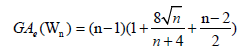Subdivision of the Cycle of the Wheel Graph Wn

In this subsection, we will give the edge version of the subdivision of a wheel graph with respect to the subdivision on the cyclic edges only. Firstly, we consider the following example.

Example 7. Let W5,1 be the graph obtained by the barycentric subdivision of the cycle of the wheel of order 5 as depicted in Figure 1. It is easy to see that the line graph of W5,1 has 22 edges. On the hand, there are 8 edges with dL(G)(μ)=dL(G))(v)=3, 8 edges of type dL(G)(μ)=3, dL(G)(v)=5, and 6 edges with dL(G)(μ)=dL(G)(v)=5.

The edge version of geometric arithmetic index of W5,1 is given as: GAe (W5,1 ) =Lemma 1. Let Wn be the wheel graph with n vertices and Wn,1 is the graph obtained after the barycentric subdivision of the cycle of the wheel. Then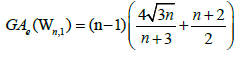Proof. It is easy to see that the line graph of Wn,1 has 4(n-1) +|Kn-1| edges. On the hand, there are 2(n – 1) edges with dL(G) (μ)=dL(G)(v)=3, 2(n-1) edges of type dL(G)(μ)=3,dL(G)(v)=n, and |Kn-1| edges with dL(G)(μ)=dL(G)(v)=n.Lemma 2. Let Wn be the wheel graph with n vertices and Wn,2 is the graph obtained after the barycentric subdivision of the cycle of the wheel. Then, the edge version of geometric arithmetic index of Wn,21 is given as:Proof. The line graph of Wn,2 has 5(n - 1) +|Kn-1| edges. On the hand, there are 2(n - 1) edges of type dL(G)(μ)=2, dL(G) (v)=3, (n–1) edges with dL(G)(μ)=dL(G)(v)=3, 2(n - 1) edges of type dL(G)(μ)=3, dL(G)(v)=n, and |Kn-1| edges with dL(G)(μ)=dL(G)(v)=n.Example 8. Let W5,3 be the graph obtained by the uniform 3-subdivision of the cycle of the wheel of order 5. ThenLemma 3. Let Wn be the wheel graph with n vertices and Wn,m is obtained after the uniform m-subdivision of the cycle of the wheel by m vertices. Then, the edge version of geometric arithmetic index of Wn,m is given as:Proof. The line graph of Wn,2 has (m+3)(n-1)+|Kn-1| edges. On the hand, there are (m-2)(n-1) edges of type dL(G)(μ)=dL(G)(v)=2, 2(n-1) edges of type dL(G)(μ)=2, dL(G)(v)=3, (n-1) edges with dL(G)(μ)=dL(G)(v)=3, 2(n-1) edges of type dL(G)(μ)=3, dL(G)(v)=n, and |Kn-1| edges with dL(G)(μ)=dL(G)(v)=n.Example 9. Let W5,2,3,4,5 be the graph obtained by the subdivision of the cycle of the wheel of order 5. ThenTheorem 1. Let Wn be the wheel graph with n vertices and the graph G=Wn,k1,k2,k3,…,kn-1 is obtained by sub diving the edges of cycle of the wheel by k1,k2,k3,…,kn-1 vertices, where ki ≥ 3 for each i=1,2,3,…,n-1. Then, the edge version of geometric arithmetic index of the graph G is given as:Proof. Since the line graph of a path Pki of length kia path of length ki-1so the line graph of Wn k1,k2,k3,…,kn-1 hasedges. On the hand, there areedges of typeedges of type dL(G) (μ)=2, dL(G)(v) = 3,

(n - 1) edges with dL(G) (μ)= dL(G)(v) = 3,2 (n - 1) edges of type dL(G) (μ)=3 , dL(G)(v) = n and |Kn-1| edges with dL(G) (μ) = dL(G) (v) = n

Since,this impliesAfter an easy simplification, we obtainLet G=Wn,k1,k2,k3,…,kn-1 be a graph obtained from the subdivision of the edges of the cycle of a wheel graph Wn of order n, where,

ki = 1, for each i=1,2,3,....,t and ki ≥ 2 for each i = t+1,t+2,....,n-1.

In fact, we can relabelled the number of edges on the cycle of the wheel so that, so that we get the desired label of the subdivision. The edge partitions of the graph G are as follows:Example 10. Let W5,1,1,2,3 be the graph obtained by the subdivision of the cycle of the wheel of order 5 as depicted in Figure 2. Then, the edge version of geometric arithmetic index of W5,1,1,2,3 is given as:Theorem 2. Let Wn,k1,k2,k3,…,kn-1 be a graph obtained from the subdivision of a wheel graph of order n, where, ki = 1 for each i=1,2,3,....,t and ki ≥ 2 for each i = t+1,t+2,....,n-1.Then, the edge version of geometric arithmetic index of Wn,k1,k2,k3,…,kn-1 is given as:Proof. From Equation 2, we have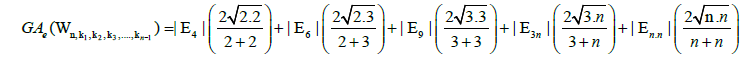Using the cardinalities of the edge partitions and after simplifications, we getSubdivision of the Spoke of the Wheel Graph Wn

In this subsection, we will give the edge version of the subdivision of a wheel graph with respect to the subdivision on the spoke of the wheel only.

Example 11. Let (W15) be the graph obtained by the barycentric subdivision of the spoke of the wheel of order 5. Then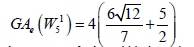Lemma 4. Let Wn be the wheel graph with n vertices and (W2n) is the barycentric subdivision of the spokes of the wheel. ThenProof. By continuing an induction argument on n, one can check that in general, the line graph of (W1n) hasedges. On the hand, there are (n-1) edges with dL(G) (μ)=3, dL(G)(v) = 4 (n-1) edges of type dL(G) (μ)=3, dL(G)(v) = n-1 and |Kn-1| edges with dL(G) (μ)=, dL(G)(v) = n-1.

So, by Equation 2, we haveAfter an easy simplification, we obtainLemma 5. Let Wn be the wheel graph with n vertices (W2n) is the uniform 2-subdivision of the spokes of the wheel. Then, the edge version of geometric arithmetic index of (W2n) is given by:Proof. By continuing an induction argument on n, one can check that in general, the line graph of (W2n) has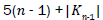edges. On the hand, there are (n-1) edges of type dL(G) (μ)= dL(G)(v) = 4,2 (n-1) edges with dL(G) (μ) = 3 & dL(G)(v) = 4,(n-1) edges of type dL(G) (μ) = 2 & dL(G)(v) = 3,(n-1) edges of type dL(G) (μ) = 2 , dL(G)(v) = n-1 and |Kn-1| edges with dL(G) (μ) = dL(G)(v) = n-1.

Since,this impliesAfter an easy simplification, we obtainTheorem 3. Let Wn be the wheel graph with n vertices Wmn is the uniform m -subdivision of the spokes of the wheel by m>2 vertices. Then, the edge version of geometric arithmetic index of Wmn is given as:Proof. It is easy to see that the line graph of Wnm hasedges. On the hand, there areedges of type dL(G) (μ) = 2 , dL(G)(v) = n-1 edges of type dL(G) (μ) = 2 , dL(G)(v) = 3 ,(n-1) edges of type dL(G) (μ) = 3 , dL(G)(v) = 4 ,(n-1) edges of type

dL(G) (μ) = dL(G)(v) = 4 , 2(n-1) edges of type dL(G) (μ) = 2 , dL(G)(v) = n-1 and |Kn-1| edges with dL(G) (μ) = dL(G)(v) = n-1.

Since,this impliesAfter simplification, we obtainArbitrary Subdivision of Wheel Graph

In this subsection, we will give the edge version of the arbitrary subdivision of a wheel graph.

Lemma 6. Let Wn be the wheel graph with n vertices and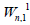is the barycentric subdivision of the wheel graph ThenProof. By continuing an induction argument on n one can check that in general, the line graph ofhasedges. On the hand, there are 4 (n-1) edges with dL(G) (μ) = dL(G)(v) = 3 ,(n-1) edges of type dL(G) (μ) = 3 , dL(G)(v) = n-1 and |Kn-1| with dL(G) (μ) = dL(G)(v) = n-1

Since,this implies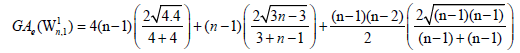After an easy simplification, we obtainLemma 7. Let Wn be the wheel graph with n vertices andis the 2-uniform subdivision of the wheel graph. ThenProof. By continuing an induction argument on n, one can check that in general, the line graph of Wn has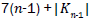edges. On the hand, there are 3 (n-1) edges withedges of typeedges of typeand |Kn-1| edges with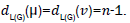Since,this impliesAfter an easy simplification, we obtainTheorem 4. Let Wn be the wheel graph with n vertices and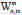is the uniform m>2 -subdivision of the wheel. Then, the edge version of geometric arithmetic index ofis given as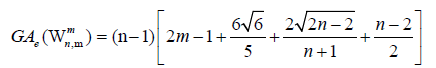Proof. By continuing an induction argument on n, one can check that in general, the line graph of Wn hasedges. On the hand, there are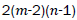edges of typeedges of typeedges of type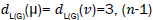edges of type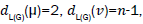and |Kn-1| edges with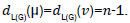Since,this impliesAfter an easy simplification, we obtainLetbe a graph obtained from the subdivision of the edges of the wheel graph Wn of order n, where, ki=1, for eachfor the edges of cycle of the wheel and li=1 for each i = 1,2,3,..., s and li≥ 2 for eachfor the spokes of the wheel.

The edge partitions of the graph G are as follows: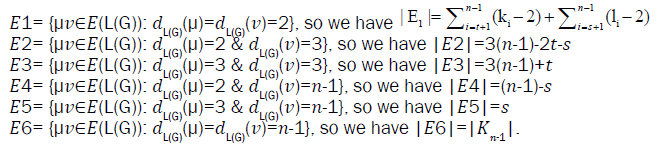Example 12. Letbe the graph obtained by the subdivision of the wheel of order 5 as depicted in Figure 3. Then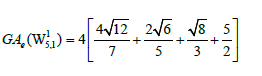Figure 3:and its line graph isin red color.

Theorem 5. Letbe a graph obtained from the subdivision of a wheel graph. Then, the edge version of geometric arithmetic index of G is given as: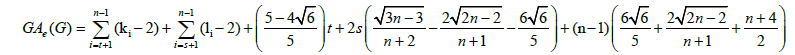Using the cardinalities of the edge partitions and after simplifications, we getConclusion

In Theoretical Chemistry, the topological indices and molecular structure descriptors are used for modeling physicochemical, toxicological, biological, and other properties of chemical compounds. In recent years, some researchers are interested in studying the topological indices of certain nanotubes and nanotori, for example, see [21,22]. In this paper, we have investigated the new version of the geometric arithmetic index of arbitrary subdivisions of a wheel graph of order n.

Acknowledgements

This research is partially supported by the University Grant, University of Sharjah, Sharjah, UAE.